# seaborn.JointGrid¶

class `seaborn.``JointGrid`(x, y, data=None, height=6, ratio=5, space=0.2, dropna=True, xlim=None, ylim=None, size=None)

Grid for drawing a bivariate plot with marginal univariate plots.

`__init__`(self, x, y, data=None, height=6, ratio=5, space=0.2, dropna=True, xlim=None, ylim=None, size=None)

Set up the grid of subplots.

Parameters
x, ystrings or vectors

Data or names of variables in `data`.

DataFrame when `x` and `y` are variable names.

heightnumeric

Size of each side of the figure in inches (it will be square).

rationumeric

Ratio of joint axes size to marginal axes height.

spacenumeric, optional

Space between the joint and marginal axes

dropnabool, optional

If True, remove observations that are missing from x and y.

{x, y}limtwo-tuples, optional

Axis limits to set before plotting.

`jointplot`

High-level interface for drawing bivariate plots with several different default plot kinds.

Examples

Initialize the figure but don’t draw any plots onto it:

```>>> import seaborn as sns; sns.set(style="ticks", color_codes=True)
>>> g = sns.JointGrid(x="total_bill", y="tip", data=tips)
```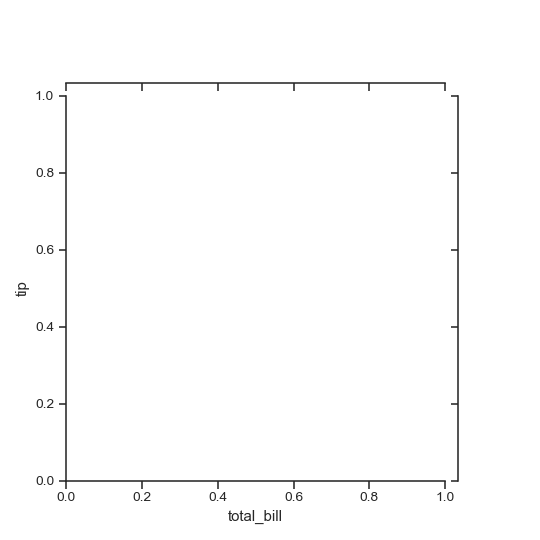```>>> g = sns.JointGrid(x="total_bill", y="tip", data=tips)
>>> g = g.plot(sns.regplot, sns.distplot)
```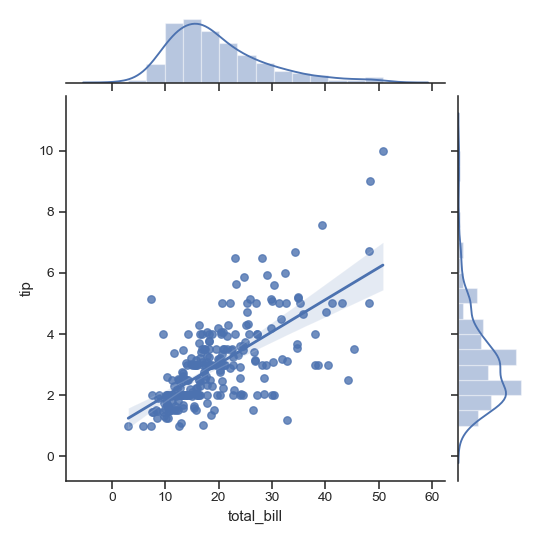Draw the join and marginal plots separately, which allows finer-level control other parameters:

```>>> import matplotlib.pyplot as plt
>>> g = sns.JointGrid(x="total_bill", y="tip", data=tips)
>>> g = g.plot_joint(sns.scatterplot, color=".5")
>>> g = g.plot_marginals(sns.distplot, kde=False, color=".5")
```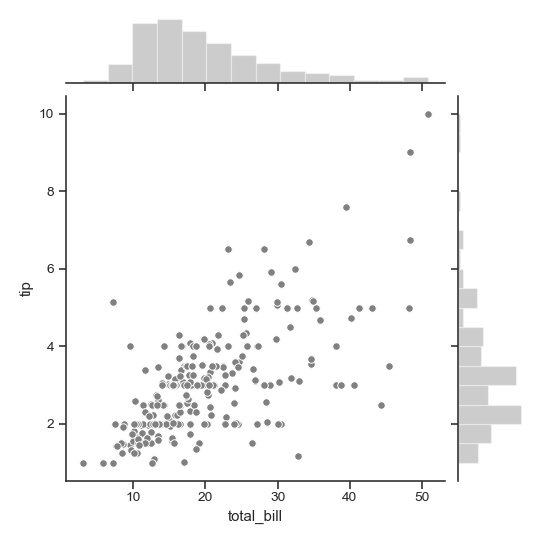Draw the two marginal plots separately:

```>>> import numpy as np
>>> g = sns.JointGrid(x="total_bill", y="tip", data=tips)
>>> g = g.plot_joint(sns.scatterplot, color="m")
>>> _ = g.ax_marg_x.hist(tips["total_bill"], color="b", alpha=.6,
...                      bins=np.arange(0, 60, 5))
>>> _ = g.ax_marg_y.hist(tips["tip"], color="r", alpha=.6,
...                      orientation="horizontal",
...                      bins=np.arange(0, 12, 1))
```Remove the space between the joint and marginal axes:

```>>> g = sns.JointGrid(x="total_bill", y="tip", data=tips, space=0)
>>> g = g.plot_joint(sns.kdeplot, cmap="Blues_d")
```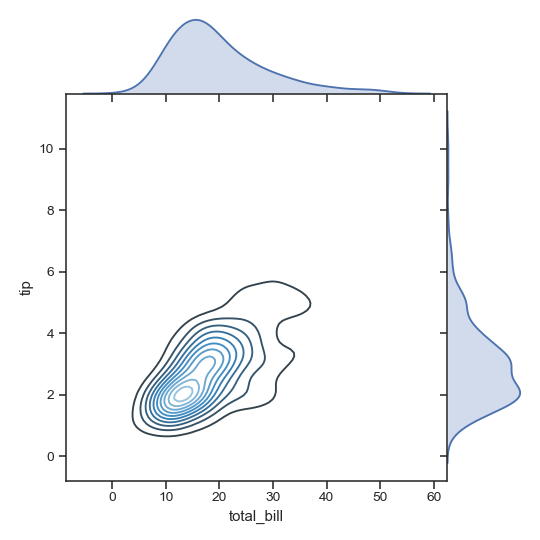Draw a smaller plot with relatively larger marginal axes:

```>>> g = sns.JointGrid(x="total_bill", y="tip", data=tips,
...                   height=5, ratio=2)
>>> g = g.plot_joint(sns.kdeplot, cmap="Reds_d")
>>> g = g.plot_marginals(sns.kdeplot, color="r", shade=True)
```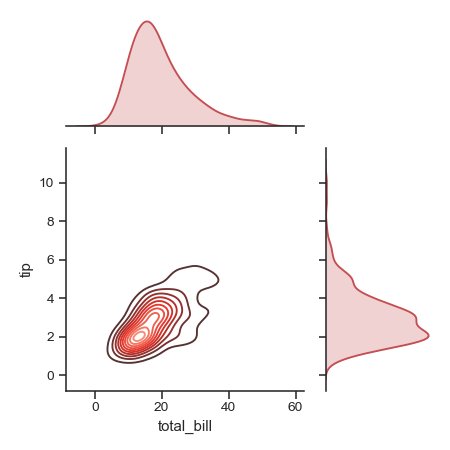Set limits on the axes:

```>>> g = sns.JointGrid(x="total_bill", y="tip", data=tips,
...                   xlim=(0, 50), ylim=(0, 8))
>>> g = g.plot_joint(sns.kdeplot, cmap="Purples_d")
>>> g = g.plot_marginals(sns.kdeplot, color="m", shade=True)
```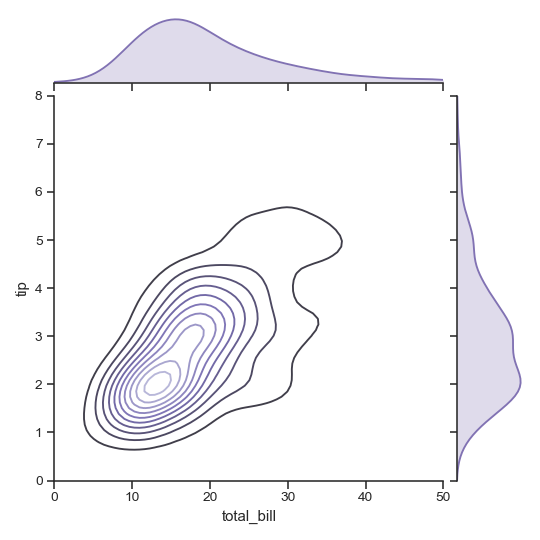Methods

 `__init__`(self, x, y[, data, height, ratio, …]) Set up the grid of subplots. `annotate`(self, func[, template, stat, loc]) Annotate the plot with a statistic about the relationship. `plot`(self, joint_func, marginal_func[, …]) Shortcut to draw the full plot. `plot_joint`(self, func, \*\*kwargs) Draw a bivariate plot of x and y. `plot_marginals`(self, func, \*\*kwargs) Draw univariate plots for x and y separately. `savefig`(self, \*args, \*\*kwargs) Wrap figure.savefig defaulting to tight bounding box. `set_axis_labels`(self[, xlabel, ylabel]) Set the axis labels on the bivariate axes.